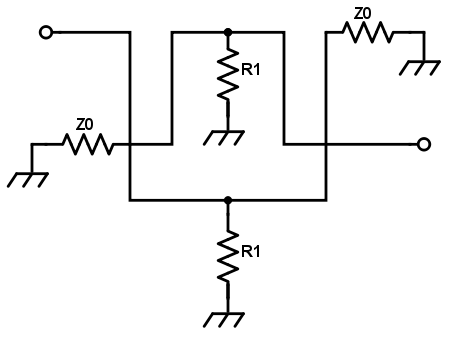# Balanced Attenuator Calculator

## This balanced attenuator calculator helps you determine the resistor (R1) values for a balanced attenuator.

(Ω) Ohm

### Overview

This calculator is designed to help calculate the value of the resistor to be used in a balanced RF attenuator. The only requirement is to enter the desired attenuation in decibels (dB) and the characteristic impedance of the transmission lines to be matched.### Equation

$$R_{1} = Z_{0}\left [\frac{1}{10^{\frac{A_{dB}}{20}}-1}\right ]$$

Where:

$$R_{1}$$ = balanced attenuator resistor

$$Z_{0}$$ = transmission line characteristic impedance

$$A_{dB}$$ = desired attenuation in dB

### Applications

An attenuator is normally passive, i.e., consisting only of a network of resistors. Its primary use is to weaken the power being supplied by a source to a level suitable for the connected load. You can say that an attenuator does the opposite of an amplifier.

In RF applications, it is normally desired to use an attenuator to weaken the signal from one transmission line to another. It can also be used to match impedances between two circuits that need to be connected. Matching impedance is important for maximum power transfer between two points in a circuit. Attenuators can also be used to isolate two different circuit stages.

The balanced attenuator is considered to be much more convenient than the other type of attenuators because you only need one value of resistance. Also, this allows the balanced topology to be used as a variable attenuator as you only need to control one parameter, R1.

Bridged-Tee Attenuator Calculator

Pi Attenuator Calculator

Reflection Attenuator Calculator